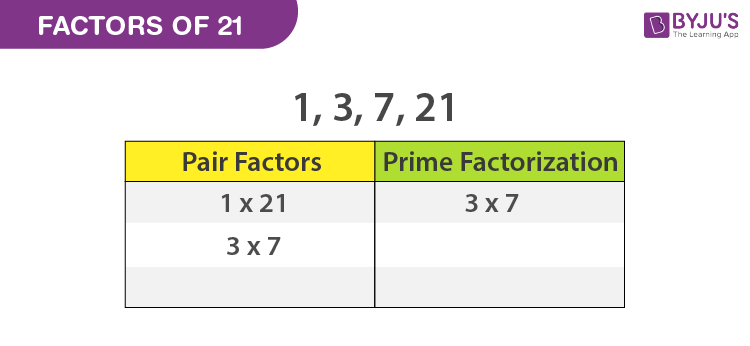# Factors of 21

The factors of 21 are the numbers that produce the result as 21 when a pair factor is multiplied together. A factor of a number divides the original number uniformly.

The factors of 21 are 1, 3, 7 and 21.

Factor pairs of the number 21 are the numbers that result in the original number multiplying them together. To find the factors of a number, 21, we can use the factorization method.## How to Find the Factors of 21?

Go through the following steps to find factors of 21.

Step 1: First, write the number 21

Step 2: Find the two numbers, which results in 21 under the multiplication, say 3 and 7, such that 3 × 7 = 21.

Step 3: We know that 3 and 7 are prime numbers with only two factors, i.e., one and the number itself.

The factors of 3 = 3 × 1

The factors of 7 = 7 x 1

So, we cannot further factorize them.

Step 4: Therefore, the factorization of 21 can be expressed as 21 = 3 × 7 × 1

Step 7: Finally, write down all the unique numbers which we can obtain from the above process.

 Factors of 21 1, 3, 7, and 21

## Pair Factors of 21

To find the pair factors of 21, multiply the two numbers to get the original number as 21. We can write both positive and negative integers in pairs as shown below:

 Positive pairs Negative pairs 1 × 21 = 21; (1, 21) (-1) × (-21) = 21; (-1, -21) 3 × 7 = 21; (3, 7) (-3) × (-7) = 21; (-3, -7) 7 × 3 = 21; (7, 3) (-7) × (-3) = 21; (-7, -3) 21 × 1 = 21; (21, 1) (-21) × (-1) = 21; (-21, -1)

Therefore, the positive pair factors of 21 are (1, 21), (3, 7), (7, 3) and (21, 1)

The negative pair factors of 21 are (-1, -21), (-3, -7), (-7, -3) and (-21, -1).

### Prime Factors of 21

As we know, 21 is a composite number, and it has prime factors. Now let us find the prime factors of 21.

• The first step is to divide the number 21 with the smallest prime number, i.e. 2.

21 ÷ 2 = 10.5

Factors should be whole numbers, so 2 cannot be the factor of 21. Hence, proceed with the next prime number, i.e. 3.

21 ÷ 3 = 7

Thus, 3 is one of the prime factors of 21.

Now, divide 7 by the numbers which are prime.

7 ÷ 2 = 3.5

7 ÷ 3 = 2.333

7 ÷÷ 5 = 1.4

7 ÷ 7 = 1

• We have received number 1 at the end of the division process; thus, we cannot proceed further.

So, the prime factors of 21 are 3 and 7, where 2 and 7 are the prime numbers.

Prime factorisation of 21 is 3 × 7.

## Video Lesson on Prime Factors### Factor of 21 – Important Facts

Some of the important facts about the factors of 21 are listed below:

• The number of factors of 21 is 4.
• The sum of all factors of 21 is equal to 32.
• The product of all factors of 21 is equal to square of 21 or 21 times of 21.

## Frequently Asked Questions on Factors of 21 – FAQs

Q1

### What are the multiples and factors of 21?

The multiples of 21 include 21, 42, 63, 84, 105, 126, 147, 168, 189, 210, etc.,
The factors of 21 are 1, 3, 7 and 21.
Q2

### What are multiples of 21?

The multiples of 21 are 21, 42, 63, 84, 105, 126, 147, 168, 189, 210, ….
Q3

### What are the factors of 21 and 24?

The factors of 21 are 1, 3, 7 and 21.
The factors of 24 are 1, 2, 3, 4, 6, 8, 12 and 24.
Q4

### Does 21 only have 2 factors?

The number 21 has 2 more factors other than 1 and 21 as it’s factors are 1, 3, 7 and 21.
Q5

### What 3 numbers make 21?

We have multiple group of 3 numbers that add up to 21 such as:
6, 7, 8 (6 + 7 + 8 = 21)
3, 8, 10 (3 + 8 + 10 = 21)
Quiz on Factors of 21